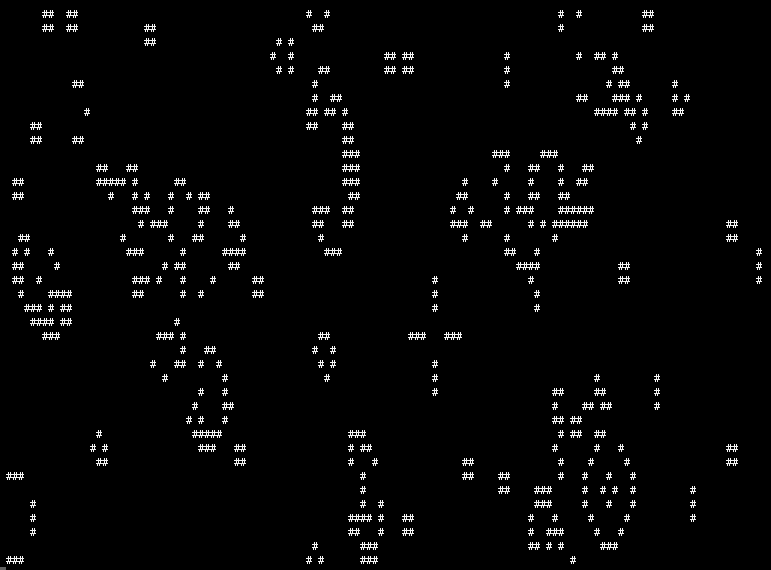John Conway's Game of Life - Go

cellular automata golang

Yet another implementation of John Conway's Game of Life in Go. I wrote this as a while back just to familiarize myself with Go. The project is maintained on GitHub: here.

Rules

1. Any live cell with fewer than two live neighbors dies, as if caused by underpopulation.
2. Any live cell with more than three live neighbors dies, as if by overcrowding.
3. Any live cell with two or three live neighbors lives on to the next generation.
4. Any dead cell with exactly three live neighbors becomes a live cell.

ScreenshotCode

package main

import (
"bytes"
"fmt"
"math/rand"
"time"
)

type Grid struct {
fg, bg [][]bool
w, h   int
}

func (grid *Grid) IsAlive(x, y int) bool {
return grid.fg[(x+grid.w)%grid.w][(y+grid.h)%grid.h]
}

func (grid *Grid) NextState(x, y int) bool {
alive := 0
for dx := -1; dx <= 1; dx++ {
for dy := -1; dy <= 1; dy++ {
if dx == 0 && dy == 0 {
// don't count self
continue
}
if grid.IsAlive(x+dx, y+dy) {
alive++
}
}
}
if grid.IsAlive(x, y) {
if alive > 3 || // die from partying too hard
alive < 2 { // die from loneliness
return false
}
return true // stay alive to party on

} else {
if alive == 3 {
return true // you're lucky, party again
}
}
return false // default, shouldn't get here
}

func (grid *Grid) Step() {
for y := 0; y < grid.h; y++ {
for x := 0; x < grid.w; x++ {
grid.bg[x][y] = grid.NextState(x, y)
}
}
// swap grids
grid.fg, grid.bg = grid.bg, grid.fg // awesome go swap
}

func (grid *Grid) String() string {
var buffer bytes.Buffer
for y := 0; y < grid.h; y++ {
for x := 0; x < grid.w; x++ {
if grid.IsAlive(x, y) {
buffer.WriteString("#")
} else {
buffer.WriteString(" ")
}
}
buffer.WriteString("\n")
}
return buffer.String()
}

func Init2dGrid(w, h int) [][]bool {
// init
grid := make([][]bool, w)
for i := range grid {
grid[i] = make([]bool, h)
}
// randomize
n := (w * h) / 2 // populate 50% of grid
for i := 0; i < n; i++ {
grid[rand.Intn(w)][rand.Intn(h)] = true
}
return grid
}

func InitGrid(w, h int) *Grid {
return &Grid {
fg: Init2dGrid(w, h),
bg: Init2dGrid(w, h),
w:  w,
h:  h,
}
}

func main() {
rand.Seed(time.Now().UTC().UnixNano()) // why on earth do you have to do this???
grid := InitGrid(128, 40)

for i := 0; i < 10000; i++ {
grid.Step()
fmt.Print("\033[H\033[2J\n")
fmt.Print(grid)
time.Sleep(time.Second / 10)
}
}


Run

Save contents in gol.go

go run gol.go